In this blog post, we are going to design a promotional game in which participants can win prizes in a campaign to promote a fictional company. We’ll see that this can be a worthwhile mental exercise. Note that most (flawed) approaches were actual promotional games we observed. Other approaches come from actual discussions I’ve had while writing this post.

We will have to comply with Dutch regulations, codified in the Burgerlijk Wetboek, Wet op de kansspelen and Gedragscode promotionele kansspelen and relevant case law (e.g. SRC College van Beroep 13 februari 2020, RB 3380; 2019/00694 - CVB). This blog focuses on analytical aspects of designing the game.

## Description of the situation

ConsumerCorp (CC) wants to celebrate the opening of its 2500th store. The company has made available 125 prizes gift cards of 10 euro to be given away during a 25-day promotional campaign (totalling 1250 euro). CC has asked us to design the mechanics for a promotional game (in the Netherlands), hence we need to comply with Dutch regulations. Our lawyer has distilled the law into three basic rules:

1. Winning should be based on chance, not on skill.
2. All prizes should be given away during the campaign.
3. The win chance should be non-zero throughout the campaign.

A marketing agency has designed a special website with a visually-attractive slot machine. When a participant visits the website, spins the slot machine and immediately sees whether he or she won a prize. Our customer is in a hurry, let’s get started right away.

## Winning at random

Our initial approach is to draw a number at random between 1 and $$n$$. If the number is equal to 1, the visitor wins a prize. Online sources report chances of winning of similar promotional actions is estimated at 1 in 400, hence we set $$n = 400$$.

Does this simple solution pass our criteria? By definition, this is a game of chance. However, the other two constraints are not guaranteed. If the number of visitors is lower than expected, not all prizes may be given away. If there are more visitors, the chance of winning may be zero at the end of the game.

Note that letting the $$n$$th visitor win is a stateful variation, where we assume the empirical mean to be the expected value (Law of Large Numbers). It, therefore, suffers the same deficiencies as the initial approach.

## Forecasting Visitors

The previous solution is only problematic if the total number of visitors is unknown. What if we accurately forecast the number of visitors, e.g. using state-of-the-art Recurrent Neural Networks (RNN) with an attention mechanism? We could build a predictive model that incorporates autoregressive features from the time series and minimize the error with a gradient-based optimization scheme.

This sounds sexy - to some of us - but what performance can we expect from such a model?

In 2018, Google hosted a research competition on Kaggle for forecasting web traffic on Wikipedia. In total, 1,095 teams participated. The first place solution reached a Symmetric mean absolute percentage error (SMAPE) of 35%, following the equation:

$\text{SMAPE}={\frac{100\%}{n}}\sum _{t=1}^{n}{\frac{\left|F_{t}-A_{t}\right|}{(|A_{t}|+|F_{t}|)/2}}$

We could have reached the same conclusion without resorting to empirical validation. There is no practical way to guarantee the visitors count, as we would need to anticipate near-infinite conditions, such as when a social media campaign is launched or the campaign will be picked up by news media.

Pursuing solutions that depend on exact visitor counts seem infeasible, both in terms of prediction error as in computational and labour costs. We conclude that our model should not use this fine-grained visitor information to guarantee compliance with the criteria. We will have to come up with some other strategy…

## Where is the time?

A logical next step would be to consider time as a variable for our model. To guarantee that there is a chance to win throughout the campaign, we distribute the prizes over the total time period.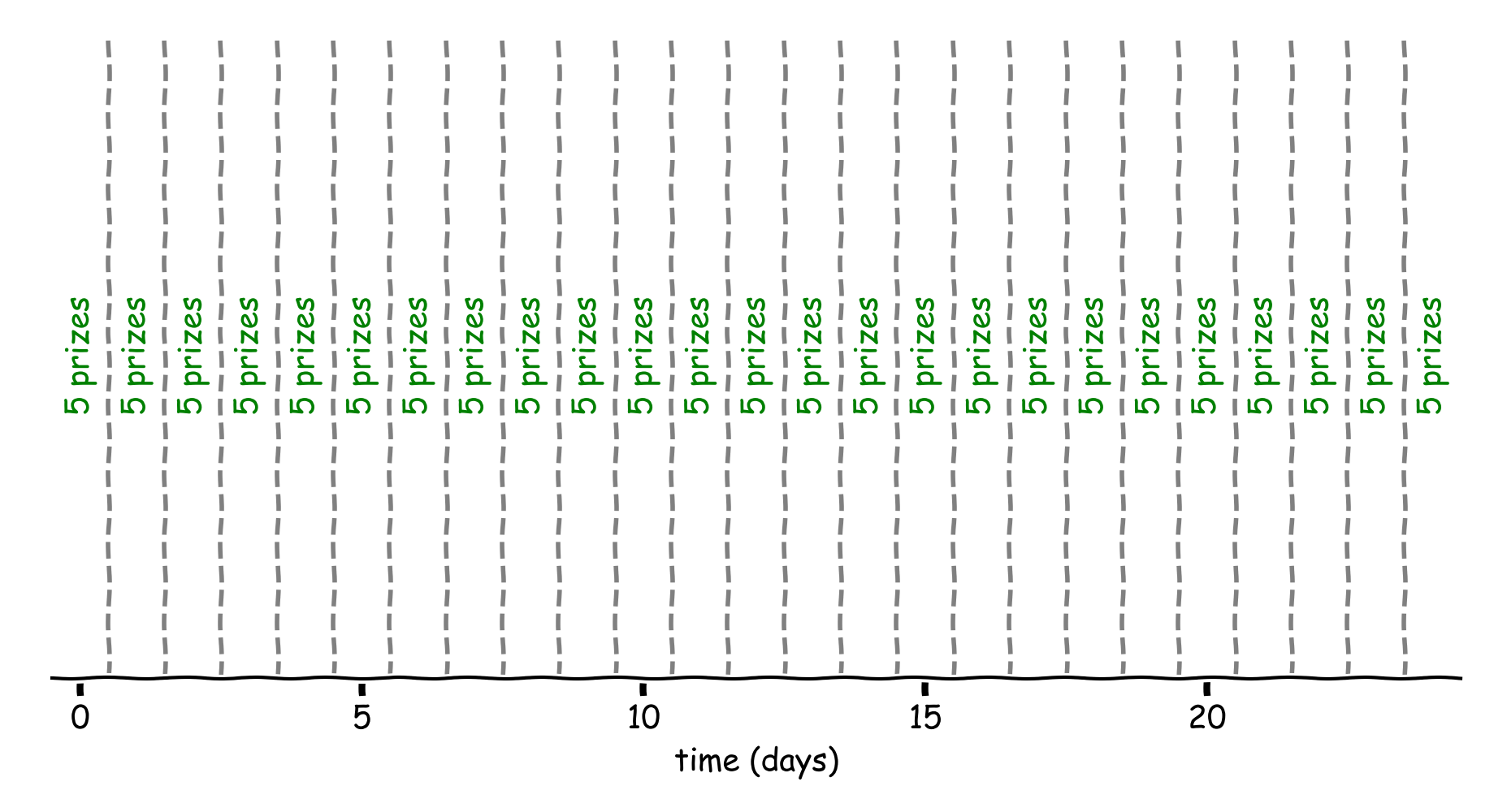Figure 1: 5 prizes a day for 25 days. Each block between two dashed lines represents a day.

Conveniently, the prizes can be precisely divided by the number of days, leaving five prizes to be distributed over each day. Every 4 hours and 48 minutes a prize. We choose the winning condition to be that the first participant to pull the lever of the slot machine after that time wins.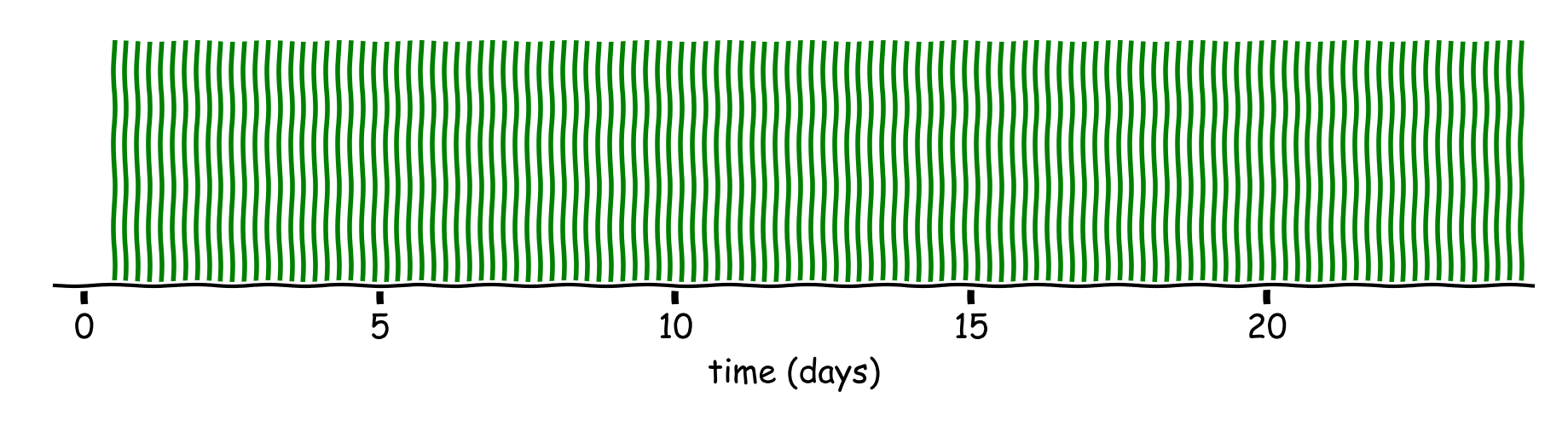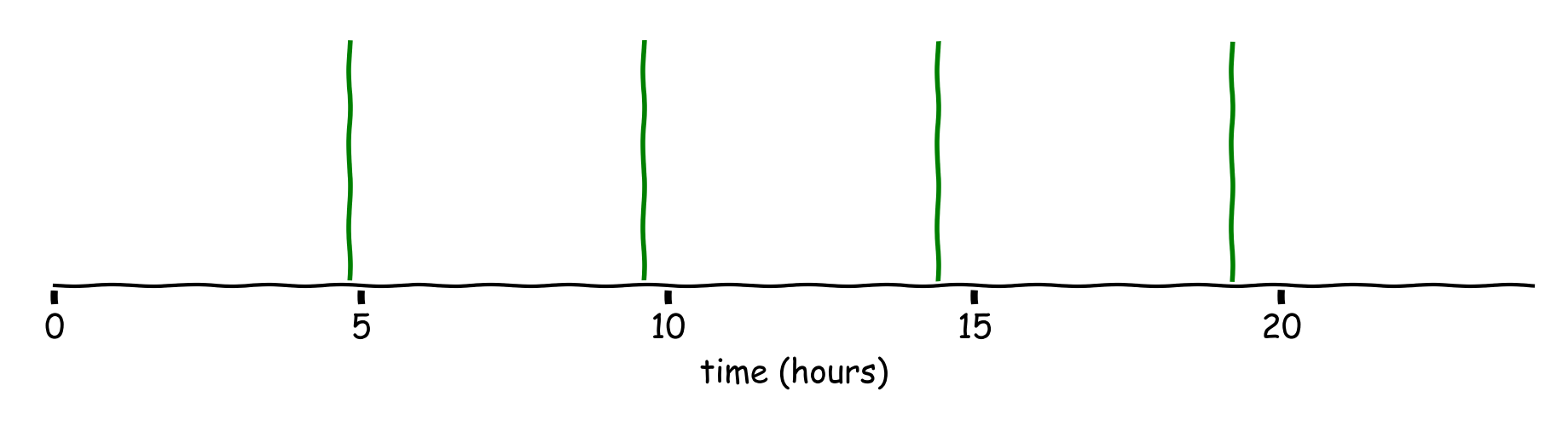Figure 2: Each green line represents a time at which a prize can be won (top) the view for the full campaign (bottom) the model zoomed to one day.

This satisfies the criteria (2) and (3)1, but not criteria (1). A clever participant will soon see the pattern and only participate at specific times. The game is not a game of chance anymore.

## Randomized time

We make the process stochastic by randomly picking a time slot within the uniform ranges (e.g. $$[0, 4h48m)$$, $$[4h48m, 9h36m)$$). The first visitor after that time wins.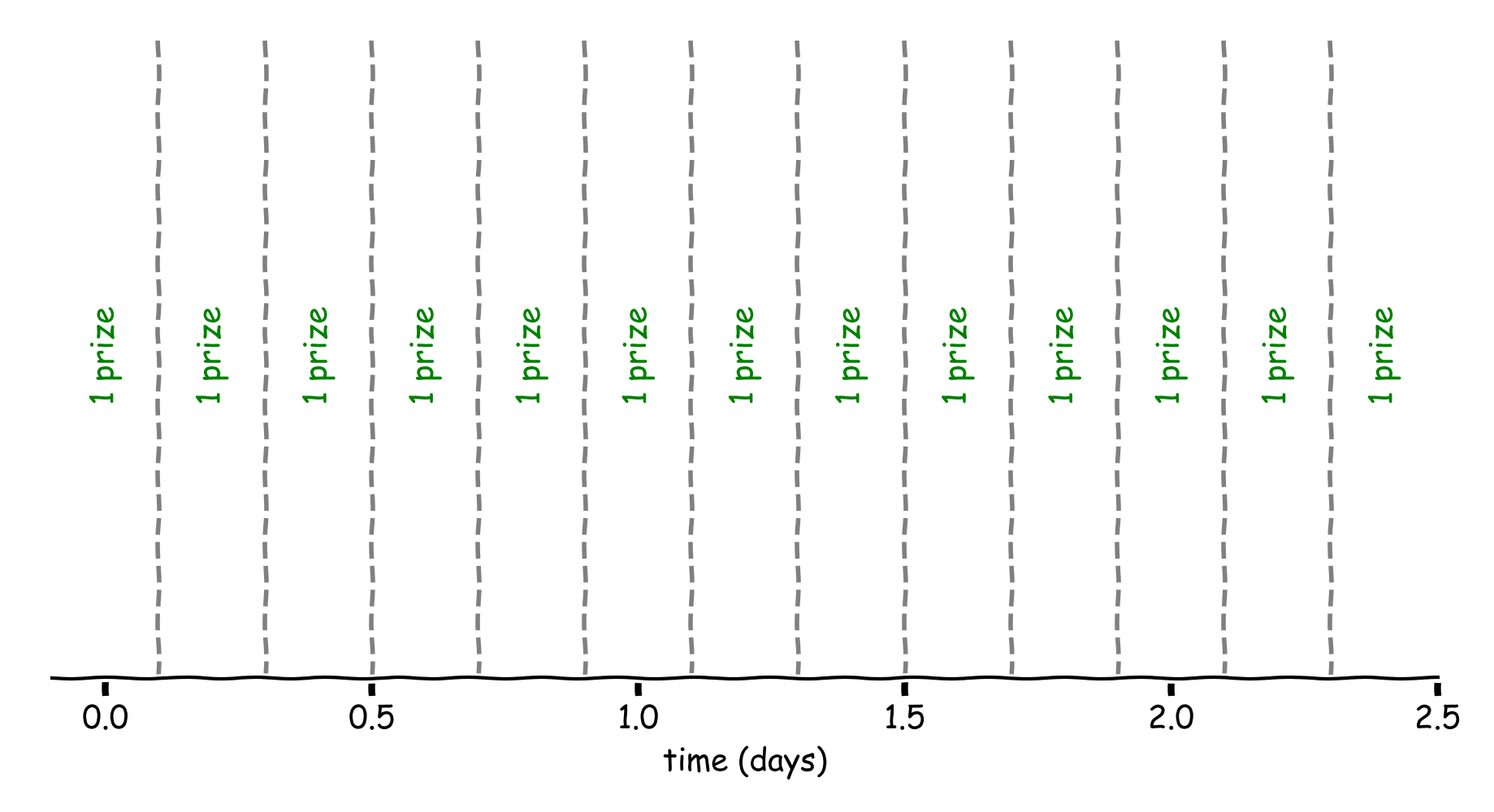Figure 3: 1 prize every 4h48m. Otherwise the same situation as before.Figure 4: The randomized model.

This solution meets all the criteria, as it ensures participants can win throughout the promotional campaign, there is an element of chance and all prizes are given away.

## Hour of day

If we inspect the previous solution closely, we can see still some margin for skilled participants to gain an advantage. If the participant is aware we are handing out prizes in this manner (white box), he or she might participate at night, when there are fewer participants.

The company has provided the visitor statistics from the past month and we observe that sessions are stable over time 2. Figure 5 shows visitor counts for each hour of the day over two different time periods.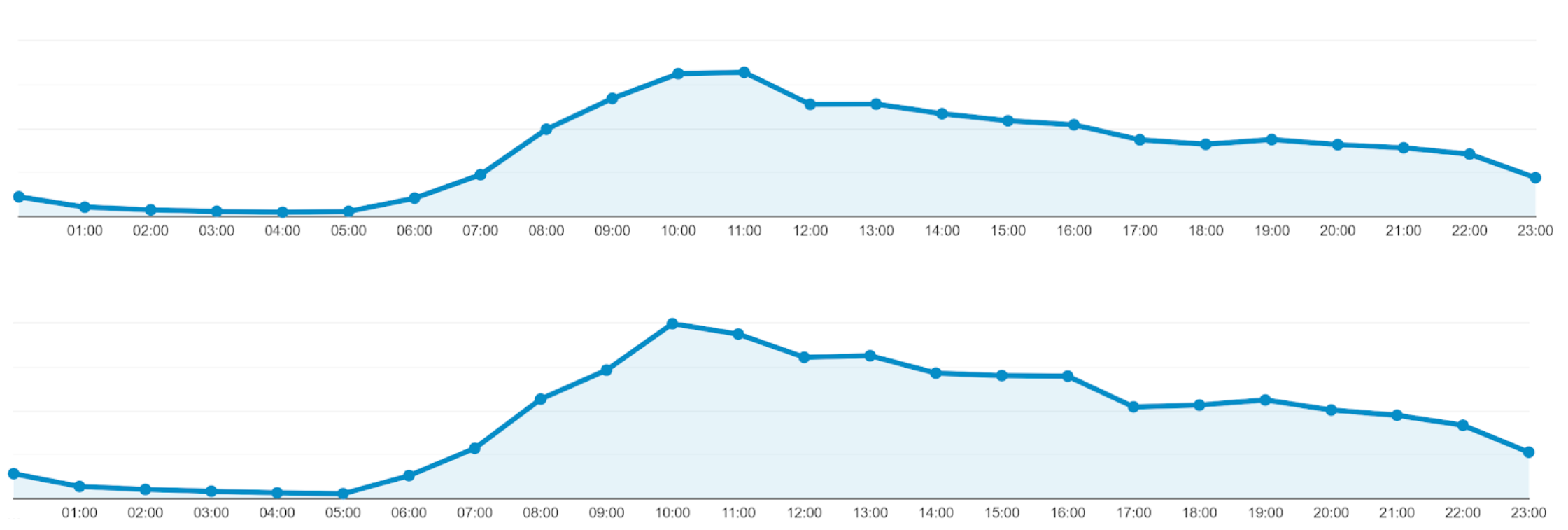Figure 5: Visitor counts for each hour of the day at different time periods. (top) 2 year of data (bottom) 1 year of data.

You may wonder: previously we saw that visitor statistics could not be used to parametrize our model, why is that not a problem here? There are multiple reasons for this, first, the current model by design satisfies criteria (2) and (3), hence we’re only constrained by criteria (1). Furthermore, finding the proportion of visitors spread over the day is a simpler problem (i.e. has a lower intrinsic dimension).

How do we parametrize the model? We cut these in five bins with approximately equal sum of visitors3. If you are familiar with complexity theory in computer science, you might recognize this as an optimization version of the Partition Problem (which is NP-hard). When working at scale, this may be a trigger, however, we are working with a small-sized problem. Brute forcing the solution involved checking $$24^4 = 331776$$ solutions.

Additionally, there are constraints that reduce the search space: we restrict to binning adjacent hours of the day and know the number of non-empty bins to be five. More precise, we need to find four edges $$0 < e_1 < e_2 < e_3 < e_4 < 24$$ where the bins are $$[0, e_1)$$, $$[e_1, e_2)$$, $$[e_2, e_3)$$, $$[e_3, e_4)$$, $$[e_4, 24)$$. With this formulation, brute forcing the solution would take $$8855$$ checks.

We minimize the mean absolute error. The solution with the lowest error is:

$$[0, 10)$$, $$[10, 12)$$, $$[12, 15)$$, $$[15, 19)$$, $$[19, 23)$$

The SMAPE score for this solution is 3.97%. Pretty good!Figure 6: the visitors per hour divided into five approximately equal bins. The dashed lines show the edges of the bins.

Residing in brute-forcing is somewhat unsatisfactory. A more elegant solution would be to perform quantile-based discretization4. Our data consists of aggregated visits (counts). We obtain the visits by repeating each observation based on the counts. To define five bins, we need to find four edges. We set these edges to be at the quintiles: the 20th, 40th 60th and 80th quantile. In computer science, finding the $$k$$th smallest number is known as a selection algorithm, which can be used to compute the quantiles. The quantile-based discretization obtains the same solution as before.

## Conclusion

We have explored possible approaches for organizing a promotional game. We have seen why some real-life approaches are flawed, and recommended a strategy that complies with our initial criteria.

The analysis explored the effect of observable variables time, visitors on the latent variable of winning and provided constant homogeneous prizes. Future analysis could extend the solution by incorporating a main prize with a mixture of other prizes. Furthermore, we would like to include simulations.

Did you come up with a solution not mentioned here, found an error or a typo or are you working in this domain? I’d be happy to discuss. I’m not a lawyer. For legal advice, I refer to a legal professional.

Notes

1. The fictional company’s website is popular. We can assume that there enough daily visitors.

2. For completeness, we should verify the hypothesis that the distribution is stable over time (e.g. Kolmogorov-Smirnov test).

3. By starting a day at midnight, we disregard the cyclic nature of our data. In this specific case, shifting the data does not provide us with a better solution.

4. There is a comparable Python implementation available in the pandas library.